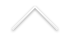Título: Sparse Markov models for high-dimensional inference

Palestrante: Guilherme Ost (Instituto de Matemática, UFRJ)
Data: 16/09/2022
Horário: 15:00h
Local: Sala C-116

Resumo: Consider a sample of size n of a finite order Markov chain. In this full generality, we can only estimate the parameters of the Markov chain (the order d and the transition probabilities) in the regime d = O(log(n)), limiting the practical application of these chains to small orders only. In this talk, we will discuss a way to overcome this constraint in a large subclass of Markov chains, namely the Mixture of Transition Distribution (MTD) models. In our main result, we will show that it is possible to select a priori the portion of the past that is relevant for the transition probabilities of a MTD, allowing the estimation of the model parameters even in the regime d = O(n). The practical performance of our estimation procedure will be illustrated through simulations. This is a joint work with Daniel Takahashi (Brain Institute - UFRN).

Sparse Markov models for high-dimensional inference